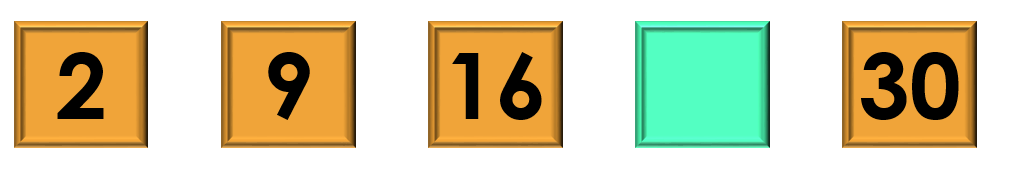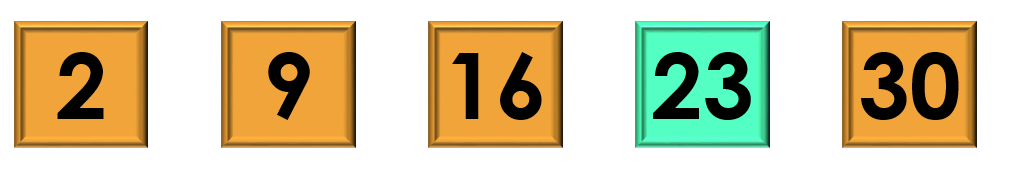1
visibility

Consider the given number pattern and identify the missing number.• A

23

• B

24

• C

25

• D

26

The correct answer is 23.

Solution:

Considering the given number pattern.Here, the numbers are increasing.
So, to find the missing number, we first find the rule.

Note that:
2 + 7 = 9
9 + 7 = 16
16 + 7 = 23
23 + 7 = 30

So, the rule is "add 7".Then, the missing number is 23.